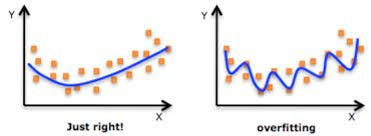The image above is great visualization of the need for regularization. The right most shows overfitting where the model is well fit to the particular dataset. The left most is more generalized, which is what we are looking for. Through the use of regularization, the model can look more like the left most graph.

‘Regularization is a way to avoid overfitting by penalizing high-valued regression coefficients. In simple terms, it reduces parameters and shrinks (simplifies) the model.’ — as defined by StatisticHowTo

Regularization is a great technique data scientists can use to avoid overfitting. Overfitting is when the model is so well trained on the training data that it is unable to pick up on trends of the validation and/or test data set. With overfitting, the model is unable to generalize, creating more complexity and high variance. When we utilize techniques to combat overfitting, we can reduce the complexity and the variance thereby increasing the performance of the test set of new and unknown data.

There are other methods to prevent overfitting like cross validation and increasing the sample size of the data, but for now let’s focus on regularization.

Regularization is sort of a family of techniques. They all do the same thing, work to reduce the complexity of a model. A model is deemed more complex when the coefficients bear significantly large weights. For example, in the medical field, we know that smoking can lead to lung cancer. If we are creating a classification model to predict whether a patient has lung cancer, we would probably make the assumption that if the person was a smoker they would have a greater chance of having lung cancer, making the weight of that variable larger. Regularization is a mathematical solution to these high coefficients that increase the model’s complexity. It works by adding a penalty to the coefficients — this penalty is dependent on the regularization method you choose — and in turn ends up gravely affecting the larger weights making them lower and less significant.

In the regularization family there are 3 particular techniques known as Lasso, Ridge, and Elastic Net. Each will involve the utilization of alpha, a tuning parameter — often a small number — multiplied to each of the coefficients. Here is the difference between them:

Lasso, or l1 regularization, harnesses the power of the absolute value in its formula. By doing so, it can shrink a parameter down to zero, thereby eliminating it in the equation. The fewer the parameters, ideally the less complex a model. We would consider using this technique when there are only a few highly significant predictors/variables. This is also a means of feature selection. Feature selection helps to identify the strongest features and eliminate the weakest to make the model less complex.

Ridge, or l2 regularization, in its formula will square the parameters resulting in a greater penalty for the higher coefficients. You can remember the difference between l1 and l2 because l2 has a ‘2’ in it and ridge squares the coefficients. Using ridge will lead to the shrinkage of the coefficients — closer to but not actually to 0 — and a reduction of the complexity. We would consider using this technique when there are large coefficients that are of similar values.

Elastic Net is a combination of both Lasso and Ridge. There is a special addition here in the formula, which is rho. Rho helps us to determine the balance between the two methods. For those of you familiar with classification metrics, f1 score to precision and recall is kind of like elastic net to ridge and lasso regression.

In sklearn, within the linear_regression module, each of these techniques have their own classes. They are fairly easy to implement thanks to this. It is very important to remember to scale your data beforehand! This is how to do it in code:

`from sklearn.preprocessing import StandardScalerscale = StandardScaler()X_train_scale = scale.fit_transform(X_train)X_test_scale = scale.transform(X_test) #NEVER FIT THE TEST DATAfrom sklearn.linear_model import Ridge, Lasso, ElasticNet#creating an instance of the ridge class & setting alpharidge_model = Ridge(alpha = .5)ridge_model.fit(X_train_scale, y_train)#######SAME THING FOR LASSO BUT FOR ELASTIC IT GOES LIKE THIS#creating an instance of elastic & setting alpha and #l1_ratio. With l1_ratio the default is .5, the higher #the number, the more l1(Lasso) in the balance. elastic_model = ElasticNet(alpha = .6, l1_ratio = .5)elastic_model.fit(X_train_scale, y_train)`

In summation, regularization is a powerful technique utilized in data science to combat the overfitting problem. This group of techniques aim to reduce the complexity of the model by shrinking the coefficients. Lasso, or l1, should be used when you have a few large coefficients. This will help to eliminate coefficients to 0. Ridge, or l2, should be used when you have a lot of large coefficients relatively the same size. This will help to lower the magnitude of the coefficients. Elastic Net is a harmonic version of regularization incorporating both l1 and l2 (depending on the ratio you specify). They are extremely easy to use thanks to the power of sklearn.

References

Definition — https://www.statisticshowto.com/regularization/

SKLearn Documentation — https://scikit-learn.org/stable/modules/classes.html#module-sklearn.linear_model

## More from Zachary Greenberg

Data Scientist / Singer